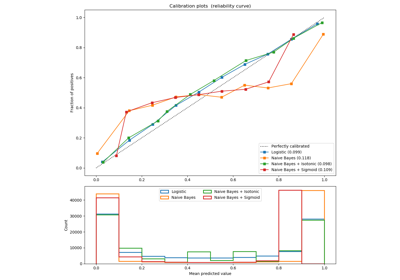# sklearn.calibration.calibration_curve¶

sklearn.calibration.calibration_curve(y_true, y_prob, normalize=False, n_bins=5, strategy='uniform')[source]

Compute true and predicted probabilities for a calibration curve.

The method assumes the inputs come from a binary classifier.

Calibration curves may also be referred to as reliability diagrams.

Read more in the User Guide.

Parameters
y_truearray, shape (n_samples,)

True targets.

y_probarray, shape (n_samples,)

Probabilities of the positive class.

normalizebool, optional, default=False

Whether y_prob needs to be normalized into the bin [0, 1], i.e. is not a proper probability. If True, the smallest value in y_prob is mapped onto 0 and the largest one onto 1.

n_binsint

Number of bins. A bigger number requires more data. Bins with no data points (i.e. without corresponding values in y_prob) will not be returned, thus there may be fewer than n_bins in the return value.

strategy{‘uniform’, ‘quantile’}, (default=’uniform’)

Strategy used to define the widths of the bins.

uniform

All bins have identical widths.

quantile

All bins have the same number of points.

Returns
prob_truearray, shape (n_bins,) or smaller

The true probability in each bin (fraction of positives).

prob_predarray, shape (n_bins,) or smaller

The mean predicted probability in each bin.

References

Alexandru Niculescu-Mizil and Rich Caruana (2005) Predicting Good Probabilities With Supervised Learning, in Proceedings of the 22nd International Conference on Machine Learning (ICML). See section 4 (Qualitative Analysis of Predictions).

## Examples using sklearn.calibration.calibration_curve¶